Specialists in Linear Flow Analysis

# Standard Configuration 11

Standard Configuration 11 is a 2D turbine profile. The off-design case for this configuration provides a good test to verify the ability of a code to solve the unsteady Navier-Stokes equations.

# Geometry

The co-ordinates of points on the profile surface can be downloaded from the here. Other details about the geometry are shown in the table below.

 Chord Length 77.8 mm 56.55 mm -40.85 degrees

There are two steady-state flow conditions defined for Standard Configuration 11: a subsonic case and a transonic off-design case, where flow separation occurs on the suction surface. The flow separates at the leading edge due to the high incident flow angle and re-attaches at approximately 30 percent chord. Only results for the off-design test case are shown here. The details of this flow condition are shown in the table below.

 Total Pressure 229.8 kPa 34 deg. 0.99

We have assumed that the total temperature is 340.0 K for the off-design test case. This is based on the fact that the experimental frequency was 211.6 Hz which corresponded to a reduced frequency of 0.309 (based on full chord). To achieve this value the reference velocity at the exit must have been approximately 334.7 m/s. The Mach number of the flow is assumed to be 0.99 which gives a total temperature of 340.0 Kelvin. The inlet Reynolds for this total temperature is 1.18 × 106.

Flow-Field Plot Cp Plot
Isentropic Mach
Number Plot
RPMTurbo Solution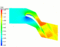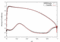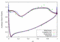[Data]

The unsteady flow response to rigid-body bending normal to chord is shown in the figures below. The reduced frequency is 0.309 (full chord) and the interblade phase angle is 180 degrees.

Cp Plot (Pressure Surface)
Cp Plot (Suction Surface)
RPMTurbo Solution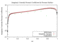[Data]

# Aerodynamic Damping versus Interblade Phase-Angle

The plot of aerodynamic damping versus interblade phase-angle for the off-design test case due to normal bending at a reduced frequency (full chord) of 0.309 is shown below.

Damping Plot RPMTurbo Data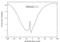[Data]